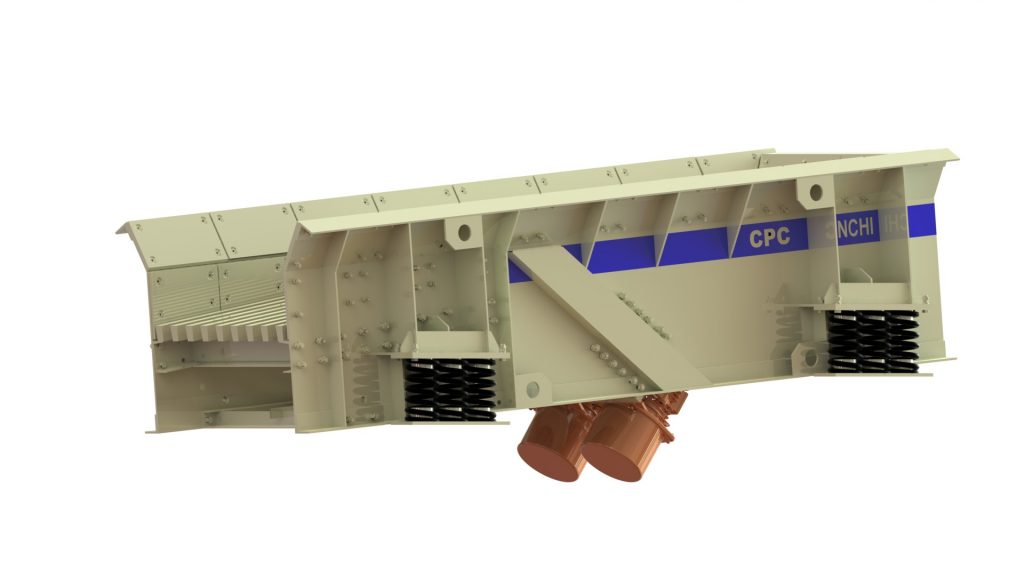Vibrating Grizzly FeederFeed Capacity

The capacity of vibrating grizzly feeders is calculated according to the following formula:

Q=3600 x f1 x f2 x f3 x L x H x V

where,

• Q=Feed Capacity in (m3/hr)
• To obtain capacity in Tons per Hour, multiply by bulk density of the material. Qt = Q x bd
• bd = 1.6 Tons/m3 for sand and crushed stone
• f1 is the Size Factor
• f1 = 1 for sand
• f1 = 0.8 to 0.9 for crushed stone up to 150mm
• f1 = 0.6 for crushed stone over 150mm
• f2 is the Moisture Factor
• f2 = 1 for dry material
• f2 = 0.8 for wet material
• f2=0.6 for sticky clay like material
• f3 is the Angle of Inclination factor
• f3=1.3 for angle of inclination = 5°
• f3=1.6 for angle of inclination = 10°
• L(m) = Width of the Vibrating Grizzly Feeder in meters
• H(m) = Height of the material layer on the table of the Vibrating Grizzly Feeder
• H = 0.2 x L for sand and crushed stone  below 40mm
• H = 0.3 x L for crushed stone upto 150mm
• H = 0.4 x L for crushed stone over 150mm
• V = Speed of the flow of material on the Vibrating Grizzly Feeder
• V depends upon
1. RPM of the Rotating Weights (i.e. RPM of the Vibro Motor or Vibrator Shaft)
2. Stroke of the Vibrating Grizzly
• Use the table below to find out the value of V
 V (m/s) = Speed of flow of material on the Vibrating Grizzly Stroke = Amplitude x 2 6mm 8mm 10mm 12mm 14mm RPM 700 0.1 0.135 0.185 0.235 0.32 750 0.135 0.175 0.215 0.255 0.335 800 0.175 0.2 0.235 0.275 0.35 850 0.205 0.225 0.265 0.29 0.36 900 0.225 0.25 0.28 0.31 0.37 950 0.25 0.27 0.3 0.325 0.375 1000 0.27 0.285 0.31 0.335 0.38

Sample Calculation for CPC Vibrating Grizzly Feeder Model VGFE 4010

Sample Process Parameters:

• Material to be Fed : Blasted Quarry Stone 100mm to 500mm, with around 10% Overburden, wet sticky clay like conditions
• Size Factor, f1 = 0.6
• Moisture Factor, f2 = 0.6

Grizzly Parameters:

• Width of Vibrating Grizzly, L = 1m
• Height of material layer on the table, H = 0.4 x L = 0.4m
• Angle of Inclination of Vibrating Grizzly = 5°
• So, f3 = 1.3
• RPM of Vibrating Grizzly:
• The RPM of the grizzly is adjustable between 750 to 1000 using a variable frequency drive.
• For Sample Calculation, let us assume the RPM to be set at 850 RPM
• Stroke of Vibrating Grizzly
• The Stroke  is adjustable between 5mm and 10mm by altering the eccentric weights of the Vibro motor
• Let us assume the Stoke to as 8mm
• By using RPM=850 and Stroke=8mm, from the table above, we get V = 0.225 m/s

So, Finally we have
f1 = 0.6
f2 = 0.6
f3 = 1.3
L = 1m
H = 0.4 x L = 0.4m
V=0.225 m/s

Using the Formula, Q=3600 x f1 x f2 x f3 x L x H x V, we get

Q=3600 x 0.6 x 0.6 x 1.3 x 1 x 0.4 x 0.225 = 151.6 m3/hr

Bulk density of Stone, bd = 1.6 Tons/m3

So, Q in Tons/Hr or TPH = 151.6*1.6 = 242.6 TPH

Hence, the capacity of CPC Vibrating Grizzly Model VGFE4010 set at 850 RPM and 8mm stroke is around 240 TPH